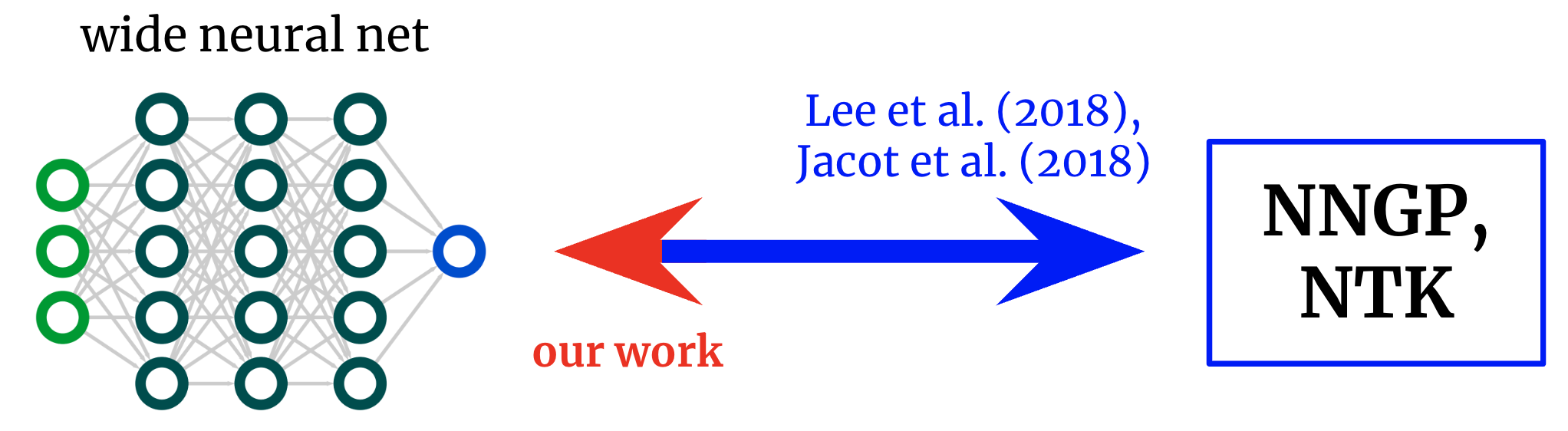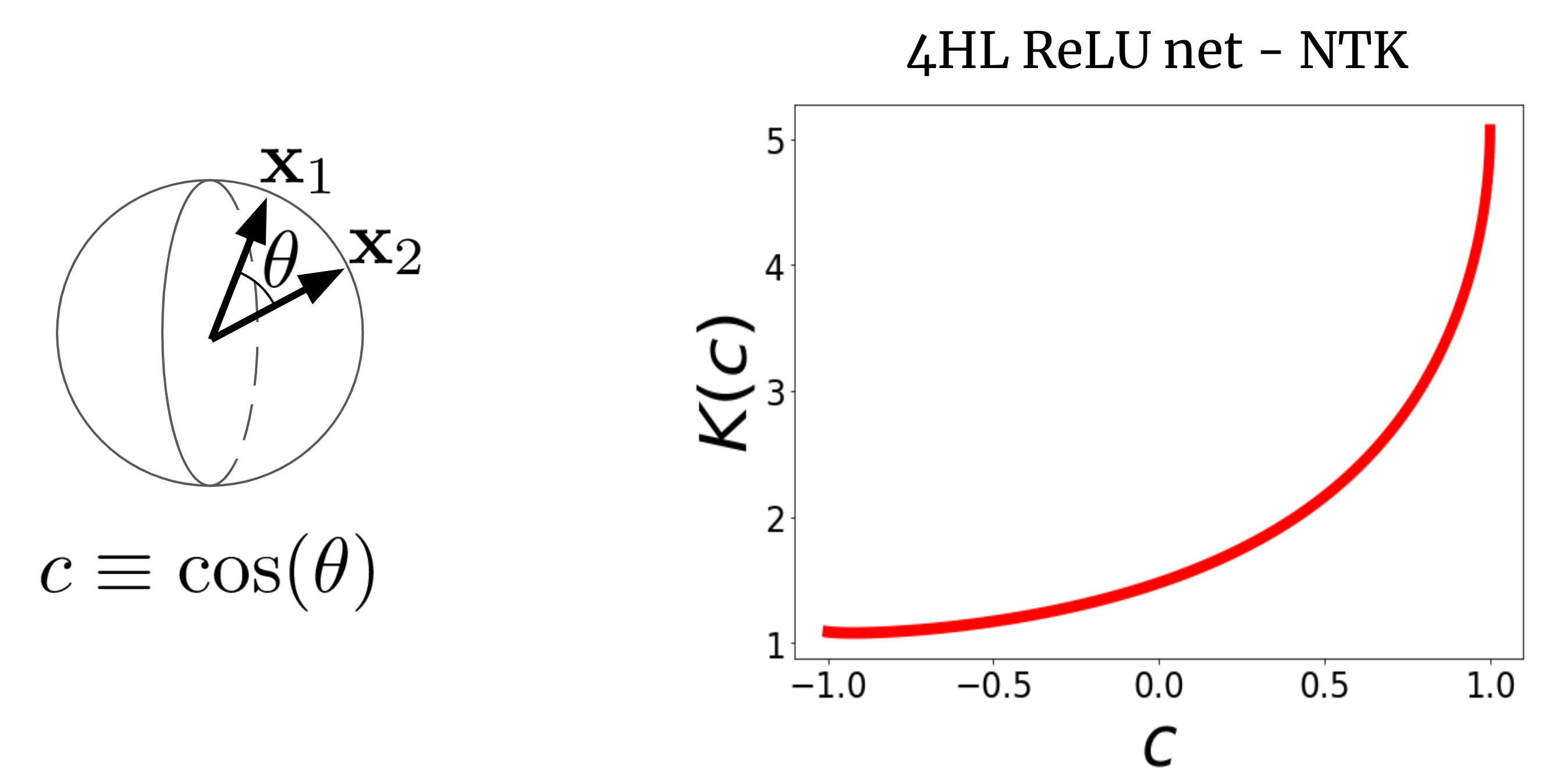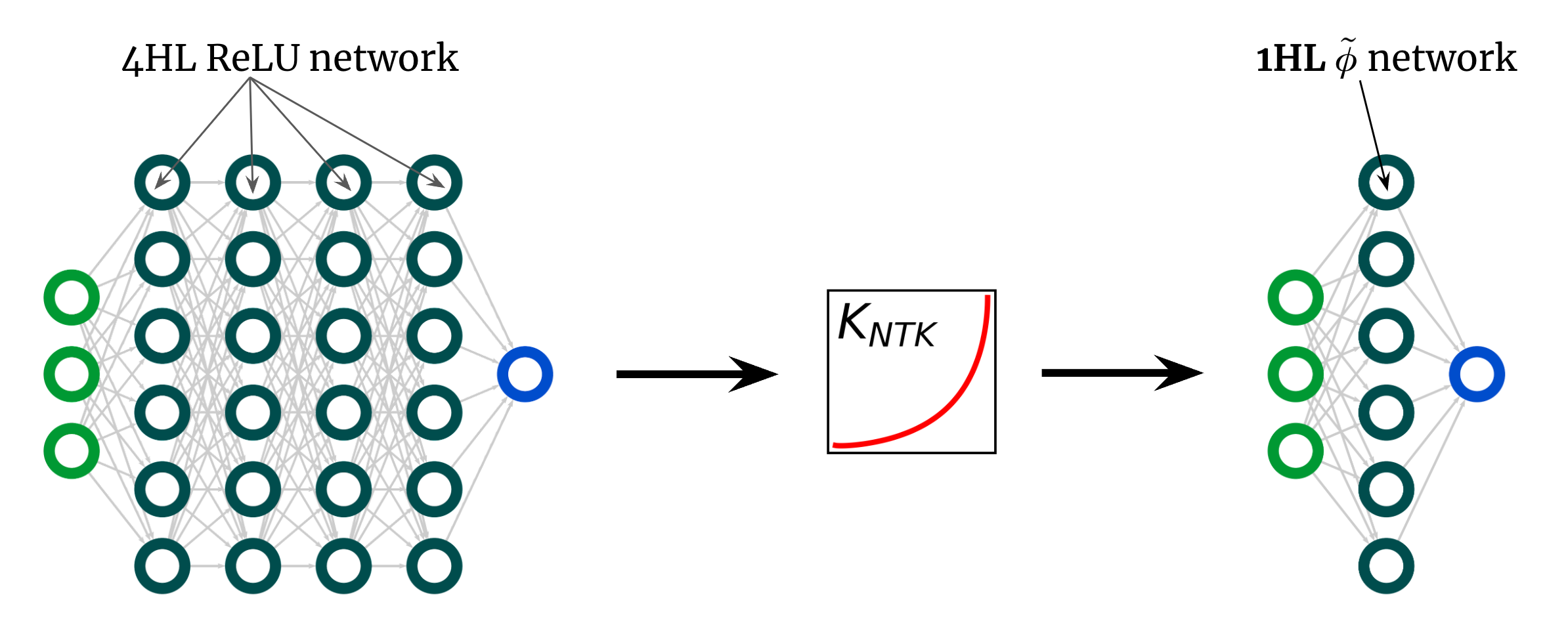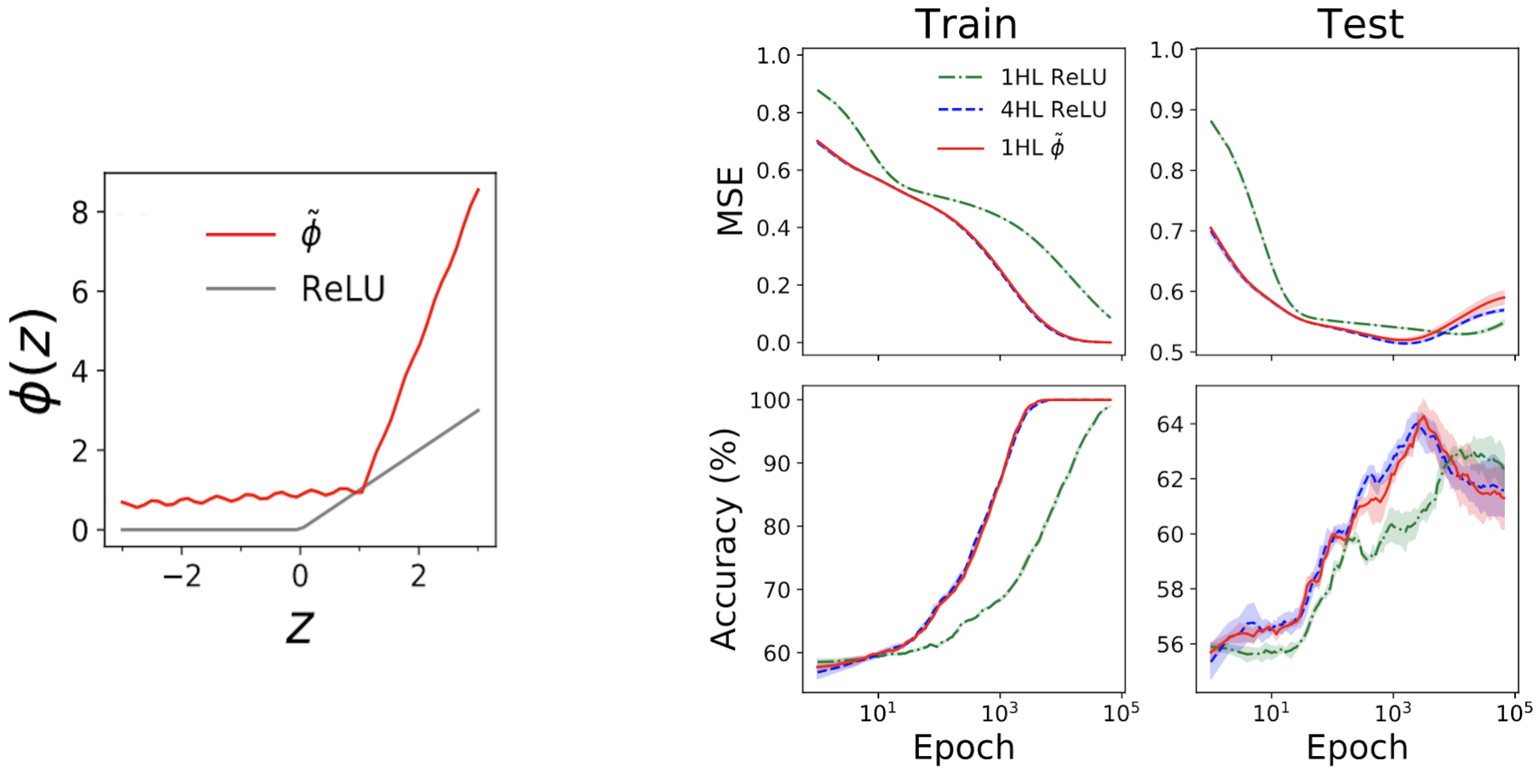# towards first-principles architecture design – The Berkeley Artificial Intelligence Research Blog

Deep neural networks have enabled technological wonders ranging from voice recognition to machine transition to protein engineering, but their design and application is nonetheless notoriously unprincipled.
The development of tools and methods to guide this process is one of the grand challenges of deep learning theory.
In Reverse Engineering the Neural Tangent Kernel, we propose a paradigm for bringing some principle to the art of architecture design using recent theoretical breakthroughs: first design a good kernel function – often a much easier task – and then “reverse-engineer” a net-kernel equivalence to translate the chosen kernel into a neural network.
Our main theoretical result enables the design of activation functions from first principles, and we use it to create one activation function that mimics deep $$\textrm{ReLU}$$ network performance with just one hidden layer and another that soundly outperforms deep $$\textrm{ReLU}$$ networks on a synthetic task.Kernels back to networks. Foundational works derived formulae that map from wide neural networks to their corresponding kernels. We obtain an inverse mapping, permitting us to start from a desired kernel and turn it back into a network architecture.

### Neural network kernels

The field of deep learning theory has recently been transformed by the realization that deep neural networks often become analytically tractable to study in the infinite-width limit.
Take the limit a certain way, and the network in fact converges to an ordinary kernel method using either the architecture’s “neural tangent kernel” (NTK) or, if only the last layer is trained (a la random feature models), its “neural network Gaussian process” (NNGP) kernel.
Like the central limit theorem, these wide-network limits are often surprisingly good approximations even far from infinite width (often holding true at widths in the hundreds or thousands), giving a remarkable analytical handle on the mysteries of deep learning.

### From networks to kernels and back again

The original works exploring this net-kernel correspondence gave formulae for going from architecture to kernel: given a description of an architecture (e.g. depth and activation function), they give you the network’s two kernels.
This has allowed great insights into the optimization and generalization of various architectures of interest.
However, if our goal is not merely to understand existing architectures but to design new ones, then we might rather have the mapping in the reverse direction: given a kernel we want, can we find an architecture that gives it to us?
In this work, we derive this inverse mapping for fully-connected networks (FCNs), allowing us to design simple networks in a principled manner by (a) positing a desired kernel and (b) designing an activation function that gives it.

To see why this makes sense, let’s first visualize an NTK.
Consider a wide FCN’s NTK $$K(x_1,x_2)$$ on two input vectors $$x_1$$ and $$x_2$$ (which we will for simplicity assume are normalized to the same length).
For a FCN, this kernel is rotation-invariant in the sense that $$K(x_1,x_2) = K(c)$$, where $$c$$ is the cosine of the angle between the inputs.
Since $$K(c)$$ is a scalar function of a scalar argument, we can simply plot it.
Fig. 2 shows the NTK of a four-hidden-layer (4HL) $$\textrm{ReLU}$$ FCN.Fig 2. The NTK of a 4HL $\textrm{ReLU}$ FCN as a function of the cosine between two input vectors $x_1$ and $x_2$.

This plot actually contains much information about the learning behavior of the corresponding wide network!
The monotonic increase means that this kernel expects closer points to have more correlated function values.
The steep increase at the end tells us that the correlation length is not too large, and it can fit complicated functions.
The diverging derivative at $$c=1$$ tells us about the smoothness of the function we expect to get.
Importantly, none of these facts are apparent from looking at a plot of $$\textrm{ReLU}(z)$$!
We claim that, if we want to understand the effect of choosing an activation function $$\phi$$, then the resulting NTK is actually more informative than $$\phi$$ itself.
It thus perhaps makes sense to try to design architectures in “kernel space,” then translate them to the typical hyperparameters.

### An activation function for every kernel

Our main result is a “reverse engineering theorem” that states the following:

Thm 1: For any kernel $K(c)$, we can construct an activation function $\tilde{\phi}$ such that, when inserted into a single-hidden-layer FCN, its infinite-width NTK or NNGP kernel is $K(c)$.

We give an explicit formula for $$\tilde{\phi}$$ in terms of Hermite polynomials
(though we use a different functional form in practice for trainability reasons).
Our proposed use of this result is that, in problems with some known structure, it’ll sometimes be possible to write down a good kernel and reverse-engineer it into a trainable network with various advantages over pure kernel regression, like computational efficiency and the ability to learn features.
As a proof of concept, we test this idea out on the synthetic parity problem (i.e., given a bitstring, is the sum odd or even?), immediately generating an activation function that dramatically outperforms $$\text{ReLU}$$ on the task.

### One hidden layer is all you need?

Here’s another surprising use of our result.
The kernel curve above is for a 4HL $$\textrm{ReLU}$$ FCN, but I claimed that we can achieve any kernel, including that one, with just one hidden layer.
This implies we can come up with some new activation function $$\tilde{\phi}$$ that gives this “deep” NTK in a shallow network!
Fig. 3 illustrates this experiment.Fig 3. Shallowification of a deep $\textrm{ReLU}$ FCN into a 1HL FCN with an engineered activation function $\tilde{\phi}$.

Surprisingly, this “shallowfication” actually works.
The left subplot of Fig. 4 below shows a “mimic” activation function $$\tilde{\phi}$$ that gives virtually the same NTK as a deep $$\textrm{ReLU}$$ FCN.
The right plots then show train + test loss + accuracy traces for three FCNs on a standard tabular problem from the UCI dataset.
Note that, while the shallow and deep ReLU networks have very different behaviors, our engineered shallow mimic network tracks the deep network almost exactly!Fig 4. Left panel: our engineered “mimic” activation function, plotted with ReLU for comparison. Right panels: performance traces for 1HL ReLU, 4HL ReLU, and 1HL mimic FCNs trained on a UCI dataset. Note the close match between the 4HL ReLU and 1HL mimic networks.

This is interesting from an engineering perspective because the shallow network uses fewer parameters than the deep network to achieve the same performance.
It’s also interesting from a theoretical perspective because it raises fundamental questions about the value of depth.
A common belief deep learning belief is that deeper is not only better but qualitatively different: that deep networks will efficiently learn functions that shallow networks simply cannot.
Our shallowification result suggests that, at least for FCNs, this isn’t true: if we know what we’re doing, then depth doesn’t buy us anything.

### Conclusion

This work comes with lots of caveats.
The biggest is that our result only applies to FCNs, which alone are rarely state-of-the-art.
However, work on convolutional NTKs is fast progressing, and we believe this paradigm of designing networks by designing kernels is ripe for extension in some form to these structured architectures.

Theoretical work has so far furnished relatively few tools for practical deep learning theorists.
We aim for this to be a modest step in that direction.
Even without a science to guide their design, neural networks have already enabled wonders.
Just imagine what we’ll be able to do with them once we finally have one.

This post is based on the paper “Reverse Engineering the Neural Tangent Kernel,” which is joint work with Sajant Anand and Mike DeWeese. We provide code to reproduce all our results. We’d be delighted to field your questions or comments.